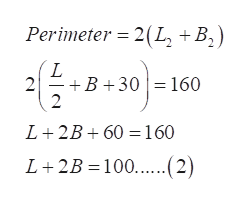# The perimeter of a rectangle is 160 meters. If a new rectangle is formed byy halving the length of one pair of opposite sides and increasing the remaining pair of sides by 30 meters each, then this new rectangle would also have a perimeter of 160 meters. What were the dimensions of the original rectangle?

Question
3 views

The perimeter of a rectangle is 160 meters. If a new rectangle is formed byy halving the length of one pair of opposite sides and increasing the remaining pair of sides by 30 meters each, then this new rectangle would also have a perimeter of 160 meters. What were the dimensions of the original rectangle?

check_circle

Step 1

Refer to the question.

Let the length and breadth of a rectangle  be ‘L’ and ‘B’ respectively. Then its perimeter is 160 m

Step 2

Now a new rectangle is formed by halving the length of one pair of opposite sides and increasing the remaining pair of sides by 30 meters each following which the perimeter of new rectangle is 160m.

That is the length is L2= L/2 and breadth B2=B+30help_outlineImage TranscriptionclosePerimeter 2(L +B2) L +B 30160 2 2 L 2B60 160 L 2B 100......(2) fullscreen
Step 3

Now solve equation 1 and equation 2 to get&n...

### Want to see the full answer?

See Solution

#### Want to see this answer and more?

Solutions are written by subject experts who are available 24/7. Questions are typically answered within 1 hour.*

See Solution
*Response times may vary by subject and question.
Tagged in

### Other